## Chapter 4 triangles R.D. Sharma Solutions for Class 10th Math MCQ's

Multiple Choice Questions

1. Sides of two similar triangles are in the ratio 4:9 . Areas of these triangles are in the ratio.
(a) 2:3
(b) 4:9
(c) 81:16
(d) 16:81

Solution

Given: Sides of two similar triangles are in the ration 4:9
To find: Ratio of these triangles
We know that the ratio of areas of two similar triangles is equal to the ratio of squares of corresponding sides.
ar(triangle 1)/ar(triangle 2) = (side 1/side 2)2 = (4/9)2
ar(triangle 1)/ar(triangle 2) = 16/81
Hence the correct answer is option d.

2. The areas of two similar triangles are in respectively 9 cm2 and 16 cm2. The ratio of their corresponding sides is
(a) 3:4
(b) 4:3
(c) 2:3
(d) 4:5

Solution

Given: Areas of two similar triangles are 9 cm2 and 16 cm2.
To find : Ratio of their corresponding sides .
We know that the ratio of areas of two similar triangles is equal to the ratio of squares of their corresponding sides .
ar(triangle1)/ar(triangle2) = (side 1/side 2)2
9/16 = (side1/side2)2
Taking square root on both sides, we get
side1/side2 = 3/4
So, the ratio of their corresponding sides is 3:4
Hence the correct answer is a.

3. The areas of two similar triangles △ABC and △DEF are 144 cm2 and 81 cm2 respectively . If longest sides of larger △ABC be 36 cm, then the longest side of the smallest triangle △DEF is
(a) 20 cm
(b) 26 cm
(c) 27 cm
(d) 30 cm

Solution

Given : Areas of two similar triangles △ABC and △DEF are 144 cm2 and 81 cm2 .
If the longest side of larger △ABC is 36 cm .
To find : the longest side of the smaller triangle △DEF
We know that the ratio of areas of two similar triangles is equal to the ratio of squares of theirs corresponding sides.
ar(△ABC)ar(△DEF) = (longest side of larger △ABC/ longest side of smaller △DEF)2
144/81 = (36/ longest side of smaller △DEF)2
Taking square root on both sides, we get
12/9 = 36/longest side of smaller △DEF
Longest side of smaller △DEF = 36 × 9/12 = 27 cm
Hence the correct answer is c .

4. △ABC and △BDE are two equilateral triangles such that D is the mid – point of BC , The ratio of the areas of triangle ABC and BDE is
(a) 2:1
(b) 1:2
(c) 4:1
(d) 1:4

Solution

Given △ABC and △BDE are two equilateral triangles such that D is the midpoint of BC.
To find : ratio of areas of △ABC and △BDE .△ABC and △BDE are equilateral triangles ; hence they are similar triangles .
Since D is the midpoint of BC, BD = DC
We know that the ratio of areas of two similar triangles is equal to the ratio of squares of the corresponding sides.
ar(△ABC)ar(△BDE) = (BC/BD)2
ar(△ABC)ar(△BDE) = (BD + DC/BD)2 [D is the midpoint of BC]
ar(△ABC)ar(△BDE) = (BD + DC/BD)2
ar(△ABC)ar(△BDE) = (2BD/BD)2
ar(△ABC)ar(△BDE) = 4/1
Hence the correct answer is c .

5. If △ABC and △DEF are similar such that 2AB = DE and BC = 8 cm, then DF =
(a) 16 cm
(b) 12 cm
(c) 8 cm
(d) 4 cm

Solution

Given : △ABC and △DEF are similar such that 2AB = DE and BC = 8 cm .
To find : EF
We know that if two triangles are similar then there sides are proportional .
Hence, for similar triangles △ABC and △DEF
AB/DE = BC/EF = CA/FD
AB/DE = BC/EF
1/2 = 8/EF
EF = 16 cm
Hence the correct answer is a .

6. In △ABC and △DEF are two triangles such that AB/DE = BC/EF = CA/FD = 2/5, then Area (△ABC) : Area (△DEF) =
(a) 2:5
(b) 4: 25
(c) 4:15
(d) 8:125

Solution

Given : △ABC and △DEF are two triangles such that AB/DE = BC/EF = CA/FD = 2/5 .
To find : ar(△ABC) : ar(△DEF)
We know that if the sides of two triangles are proportional, then the two triangles are similar.
Since AB/DE = BC/EF = CA/FD = 2/5, therefore, △ABC and △DEF are similar .
We know that the ratio of areas of two similar triangles is equal to the ratio of squares of their corresponding sides.
ar (△ABC)/ ar(△DEF) = AB2/DE2
ar (△ABC)/ar(△DEF) = 22/52
ar (△ABC)/ar(△DEF) = 4/25
Hence the correct answer is b .

7. XY is drawn parallel to the base BC of a △ABC Cutting AB at X and AC at Y. If AB = 4 BX and YC = 2 cm, then AY =
(a) 2 cm
(b) 4 cm
(c ) 6 cm
(d) 8 cm

Solution

Given : XY is drawn parallel to the base BC of a △ABC Cutting AB at X and AC at Y. If AB = 4 BX and YC = 2 cm.
To find : AYIn △AXY and △ABC ,
∠AXY = ∠B (Corresponding angles)
∠A = ∠A (common)
∴ △AXY ~ △ABC (AA similarity)
We know that if two triangles are similar , then their sides are proportional .
It is given that AB = 4BX = X.
Then, AX = 3X
AX/BX = AY/ YC
3x/1x = AY/2
AY = 3x × 2/1x
AY = 6 cm
Hence the correct answer is c .

8. Two poles of height 6 m and 11 m stand vertically upright on a plane ground . If the distance between their foot is 12 m, the distance between their tops is
(a) 12 m
(b) 14 m
(c) 13 m
(d) 11 m

Solution

Given : Two poles of heights 6m and 11m stand vertically upright on a plane ground . Distance between their foot is 2 m.
To find : Distance between their tops.
Let CD be the pole with height 6m .
AB is the pole with the height 11 m, distance between their foot i.e. DB is 12 m .
Let us assume a point EE on the pole AB which is 6 m from the base of AB.
Hence
AE = AB – 6 = 11 – 6 = 5 m
Now in right triangle AEC, Applying Pythagoras theorem
AC2 = AE2 + EC2
AC2 = 52 + 122 (Since CDEB forms a rectangle and opposite sides of rectangle are equal )
AC2 = 25 + 144
AC2 = 169
AC = 13 m
Thus, the distance between their tops is 13m.

9. In △ABC, D and E are points on side AB and AC respectively such that DE | | BC and AD : DB = 3 : 1. If EA = 3.3 cm, then AC =
(a) 1.1 cm
(b) 4 cm
(c) 4.4 cm
(d) 5.5 cm

Solution

Given : In △ABC , D and E are points on the side AB and AC respectively such that DE | | BC and AD : DB = 3:1. Also , EA = 3.3 cm
To find : AC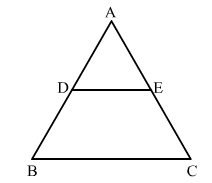In △ABC, DE | | BC,
Using corollary of basis proportionality theorem, we have
EC = 4.4 cm
Hence the correct answer is C .

10. In triangle ABC and DEF , ∠A = ∠E = 40° , AB : ED = AC : EF and ∠F = 65° , then ∠B =
(a) 35°
(b) 65°
(c) 75°
(d) 85°

Solution

Given : In △ABC and △DEF
∠A = ∠E = 40°
AB : ED = AC : EF
∠F = 65°
To find : Measure of angle B .
In △ABC and △DEF
∠A = ∠E = 40°
AB : ED = AC : EF
△ABC ~ △DEF (S.A.S Similarity crieteria)Hence in similar triangles △ABC and △DEF
∠A = ∠E = 40°
∠C = ∠F = 65°
∠B = ∠D
We know that sum of all angles of a triangle is equal to 180° .
∠A + ∠B + ∠C = 180°
40° + ∠B + 65° = 180°
∠B + 115° = 180°
∠B = 180° - 115°
∠B = 75°
Hence the correct answer is c .

11.If ABC and DEF are similar triangles such that ∠A = 47° and ∠E = 83° , then ∠C =
(a)50°
(b)60°
(c)70°
(d)80°

Solution

Given: If  ABC  and DEF are similar triangles such that
A = 47°
E = 83°
To find : Measure of angle C
In similar ABC  and DEF ,∠A = ∠D = 47°
∠B = ∠E = 83°
∠C = ∠F
We know that sum of all the angles of a triangle is equal 180° .
∠A + ∠B + ∠C = 180°
47° + 83° + ∠C = 180°
∠C + 130° = 180°
∠C = 180° - 130°
∠C = 50°
Hence the correct answer is b .

12. If D,E,F are the mid-points of sides BC,CA and AB respectively △ABC, then the ratio of triangles DEF and ABC is
(a) 1:4
(b) 1:2
(c) 2:3
(d) 4:5

Solution

Given : In △ABC, D, E and F are the midpoints of BC, CA, and AB respectively .
To find : Ratio of the areas of △DEF and △ABC
Since it is given that D and, E are the midpoints of BC, and AC respectively.
Therefore DE | | AB, DE | | FA …(1)
Again it is given that D and , F are the midpoints of BC, and, AB respectively .
Therefore, DF | | CA, DF | | AE...(2)
From (1) and (2) we get AFDE is a parallelogram.
Similarly we can prove that BDEF is a parallelogram
∠FDE = ∠A (Opposite angles of | |gm AFDE)
∠DEF = ∠B (Opposite angles of | |gm BDEF)
⇒ △ABC ~ △DEF (AA similarity criterion)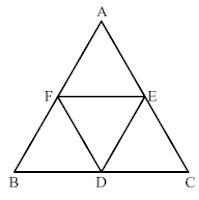We know that the ratio of areas of two similar triangles is equal to the ratio of squares of their corresponding sides .
ar(△DEF)/ar(△ABC) = (DE/AB)2
ar(△DEF)/ar(△ABC) = [(1/2(AB))/AB] (Since DE = 1/2 AB)
ar(△DEF)/ar(△ABC) = (1/4)
Hence the correct option is a .

13. In a △ABC, ∠A = 90°, AB = 5 cm and AC = 12 cm . If AD ⊥ BC, then AD =
(a) 13/2 cm
(b) 60/13 cm
(c) 13/60 cm
(d) 2√15/13 cm

Solution

Given : In △ABC ∠A = 90°, AD ⊥ BC, AC = 12 cm, and AB = 5cm
We know that the ratio of areas of two similar triangles is equal to the ratio of squares of their corresponding sides .∠C = ∠C  (Common)
∴ △ACB ~ △ADC (AA Similarity)
We got the result as b .

14. If △ABC is an equilateral triangle such that AD ⊥ BC, then AD2 =
(a) (3/2) DC2
(b) 2 DC2
(c) 3 CD2
(d) 4 DC2

Solution

Given : In an equilateral △ABC, AD ⊥ BC .
Since AD ⊥ BC, BD = CD = BC/2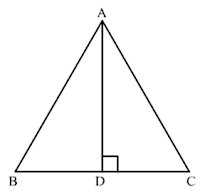BC2 = AD2 + DC2 (Since AC = BC)
(2DC)2 = AD2 + DC2 (Since BC = 2DC)
We got the result as c .

15. In a △ABC , AD is the bisect of ∠BAC . If AB = 6 cm , AC = 5 cm and BD = 3 cm, then DC =
(a) 11.3 cm
(b) 2.5 cm
(c) 3:5 cm
(d) None of these

Solution

Given : In a △ABC, AD is the bisector of ∠BAC . AB = 6 cm and AC = 5 cm and  BD = 3 cm.
To find : DC
We know that the internal bisector of angle of a triangle divides the opposite side internally in the ratio of the sides containing the angle ,
Hence ,
AB/AC = BD/DC
6/5 = 3/DC
DC = 5×3/6
DC = 2.5 cm
Hence we got the result b.

16. In △ABC, AD is the bisector of ∠BAC . If AB = 8 cm, BD = 6 cm and DC = 3 cm . Find AC
(a) 4 cm
(b) 6 cm
(c) 3 cm
(d) 8 cm

Solution

Given: In a △ABC, AD is the bisector of ∠BAC . AB = 8 cm, and DC =3 cm and BD = 6 cm .
To find : AC
We know that the internal bisector of a triangle divides the opposite side internally in the ratio of the sides containing the angle .
AB/AC = BD/DC
8/AC = 6/3
AC = 8×3/6
AC = 4 cm
Hence we got the result a.

17. ABCD is a trapezium such that BC | | AD and AD = 4 cm . If the diagonals AC and BD intersect at O such that AO/OC = DO/OB = 1/2 , then BC =
(a) 7 cm
(b) 8 cm
(c) 9 cm
(d) 6 cm

Solution

Given : ABCD is a trapezium in which BC | | AD and AD = 4 cm
The diagonals AC and BD intersect at O such that AO/OC = DO/OB = 1/2
To find : DCIn △AOD and △COB
∠ODA = ∠OBC (Alternate angles)
∠AOD = ∠BOC (Vertically opposite angles)
So, △AOD ~ △COB (AAA similarity)
Now, corresponding sides of similar △’s are proportional .
⇒ 1/2 = 4/BC
⇒ BC = 8 cm
Hence the correct answer is b .

18. If ABC is a right – angled at B and M, N are the mid – points of AB and BC respectively, then 4(AN2 + CM2) =
(a) 4 AC2
(b) 5 AC2
(c ) 5/4 AC2
(d) AC2

SolutionM is the mid – point of AB.
∴ BM = AB/2
N is the mid – point of BC .
∴ BN = BC/2
Now,
AN2 + CM2 = (AB2 + (1/2 BC)2) + ((1/2 AB)2 + BC2
= AB2 + 1/4 BC2 + 1/4 AB2 + BC2
= 5/4 (AB2 + BC2)
⇒ 4(AN2 + CM2) = 5 AC2
Hence option b is correct .

19. If in △ABC and △DEF , AB/DE = BC/FD , then △ABC ~ △DEF when
(a) ∠A = ∠F
(b) ∠A = ∠D
(c) ∠B = ∠D
(d) ∠B = ∠E

Solution

Given : In △ABC and △DEF , AB/DE = BC/FD .
We know that if in two triangles, one pair of corresponding sides are proportional and the included angles are equal , then the two triangles are similar .
Then ,
∠B = ∠D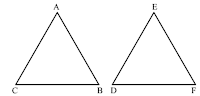Hence, △ABC is similar to △DEF , we should have ∠B = ∠D
Hence the correct answer is c.

20. If in two triangles ABC and DEF , AB/DE = BC/FE = CA/FD , then
(a) △FDE ~ △CAB
(b) △FDE ~ △ABC
(c) △CBA ~ △FDE
(d) △BCA ~ △FDE

Solution

We know that if two triangles are similar if their corresponding sides are proportional .It is given that △ABC and △DEF are two triangles such that AB/DE = BC/EF = CA/FD .
∠A = ∠D
∠B = ∠E
∠C = ∠F
∴ △CAB ~ △FDE
Hence the correct answer is a .

21. △ABC ~ △DEF, ar(△ABC) = 9 cm2 . If BC = 2.1 cm, then the measure of EF is
(a) 2.8 cm
(b) 4.2 cm
(c) 2.5 cm
(d) 4.1 cm

Solution

Given : Ar(△ABC) = 9 cm2 , Ar(△DEF) = 16 cm2 and BC = 2.1 cm
To find : measure of EF
We know that the ratio of areas of two similar triangles is equal to the ratio of squares of their corresponding sides .
Ar(△ABC)/Ar(△DEF) = BC2/EF2
9//16 = 2.12
3/4 = 2.1/EF
EF = 2.8 cm
Hence the correct answer is a .

22. The length of the hypotenuse of an isosceles right triangle whose one side is 4√2 cm is
(a) 12 cm
(b) 8 cm
(c) 8√2 cm
(d) 12√2 cm

Solution

Given : One side of isosceles right triangle is 4√2 cm
To find : Length of the hypotenuse .
We know that in isosceles triangle two sides are equal .In isosceles right triangle ABC , let AB and AC be the two equal sides of measures 4√2 cm .
Applying Pythagoras theorem, we get
BC2 = AB2 + AC2
BC2 = (4√2)2 + (4√2)2
BC2 = 32 + 32
BC2 = 64
BC = 8 cm
Hence correct answer is b .

23. A man goes 24 m due west and then 7 m due north. How far is he from the starting point ?
(a) 31 m
(b) 17 m
(c) 25 m
(d) 26 m

Solution

A man goes 24 m due to west and then 7 m due north.
Let the man starts from point B and goes 24 m due to west and reaches point A, then walked 7 m north and reaches point C.
Now we have to find the distance between the starting point and the end point i.e. BC.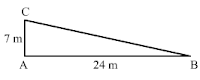In right triangle ABC , applying Pythagoras theorem we, get
BC2 ,= AB2 + AC2
BC2 = (24)2 + (7)2
BC2 = 576 + 49
BC2 = 625
BC = 25 m
Hence correct answer is c .

24. △ABC ~ △DEF . If BC = 3 cm , EF = 4 cm and ar(△ABC) = 54 cm2 , then ar(△DEF) = (a) 108 cm2
(b) 96 cm2
(c) 48 cm2
(d) 100 cm2

Solution

Given : In △ABC and △DEF
△ABC ~△DEF
Ar(△ABC ) = 54 cm2 BC = 3 cm and EF = 4 cm
To find : Ar(△DEF)
We know that the ratio of areas of two similar triangles is equal to the ratio of squares of their corresponding sides .
Ar(△ABC )/Ar(△DEF) = BC2/EF2
54/Ar(△DEF) = 32/42
54/ Ar(△DEF) = 9/16
Ar(△DEF) = 16×54/9
Ar(△DEF) = 96 cm2
Hence the correct answer is b .

25. △ABC ~△PQR such that ar(△ABC) = 4 ar(△PQR). If BC = 12 cm, then QR =
(a) 9 cm
(b) 10 cm
(c) 6 cm
(d) 8 cm

Solution

Given : In △ABC and △PQR
△ABC ~△PQR
Ar(△ABC ) = 4Ar (△PQR)
BC = 12 cm
To find : Measure of QR
We know that the ratio of areas of two similar triangles is equal to the ratio of squares of their corresponding sides .
Ar(△ABC )/Ar(△PQR) = BC2/QR2
4 Ar(△PQR)/ Ar(△PQR) = 122/QR2 (Given Ar(△ABC ) = 4 Ar(△PQR))
4/1 = 122/QR2
2/1 = 12/QR
QR = 6 cm
Hence the correct answer is c .

26. The areas of two similar triangles are 121 cm2 and 64cm2 respectively .If the median of the first triangle is 12.1 cm, then the corresponding median of the other triangle is
(a) 11 cm
(b) 8.8 cm
(c) 11.1 cm
(d) 8.1 cm

Solution

Given : The area of two similar triangles is 121 cm2 and 64 cm2 respectively . The median of the first triangle is 2.1 cm . To find : Corresponding medians of the other triangle
We know that the ratio of areas of two similar triangles is equal to the ratio of squares of their medians .
ar(triangle1)/ar(triangle2) = (median1/median2)2
121/64 = (12.1/median2)2
Taking square root on both side, we get
11/8 = 12.1 cm/median2
⇒ median2 = 8.8 cm
Hence the correct answer is b .

27. In an equilateral triangle ABC if AD ⊥ BC, then AD2 =
(a) CD2
(b)2CD2
(c) 3CD2
(d) 4CD2

Solution

In an equilateral △ABC , AD ⊥ BC .In △ADC, applying Pythagoras theorem , we get
BC2 = AD2 + DC2 (∵BC = 2DC)
Hence the correct option is c.

28. In an equilateral triangle ABC if AD ⊥ BC, then

Solution

△ABC is an equilateral triangle and AD ⊥ BC .
In △ABD, applying Pythagoras theorem, we get
AB2 = AD2 + (1/2BC)2 (∵ BD = 1/2 BC)
AB2 = AD2 + (1/2 AB)2 (∵ AB = BC)
AB2 = AD2 + 1/4 AB2
We got the result as b .

29. △ABC is an isosceles triangle in which ∠C = 90 . If AC = 6 cm, then AB =
(a) 6 √2 cm
(b) 6 cm
(c) 2√6 cm
(d) 4√2 cm

Solution

Given : In an isosceles △ABC , ∠C = 90° . If AC = 6 cm .
To find : AB
In an isosceles △ABC, ∠C = 90° .
Therefore , BC = AC = 6 cm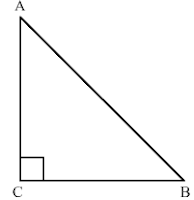Applying Pythagoras theorem in △ABC , we get
AB2 = AC2 + BC2
AB2 = 62 + 62 (AC = BC, sides of isosceles triangle)
AB2 = 36 + 36
AB2 = 72
AB = 6√2 cm
We got the result as a.

30. If in two triangle ABC and DEF , ∠A = ∠E, ∠B = ∠F, then which of the following is not true?
(a) BC/DF = AC/DE
(b) AB/DE = BC/DF
(c) AB/EF = AC/DE
(d) BC/DF = AB/EF

Solution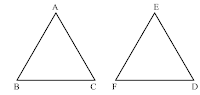In △ABC and △DEF
∠A = ∠E
∠B = ∠F
∴ △ABC and △DEF are similar triangles.
Hence AB/EF = BC/FD = CA/DE
Hence the correct answer is b .

31 . In the given figure the measure of ∠A and ∠F are respectively
(a) 50°, 40°
(b) 20°, 30°
(c) 40°, 50°
(d) 30°, 20°Solution

△ABC and △DEF ,
AB/AC = EF/ED
∠A = ∠E = 130°
△ABC ~ △EFD (SAS Similarity)
∴ ∠F = ∠B = 30°
∠D = ∠C = 20°
Hence the correct answer is b .

32. In the given figure , the value of x for which DE | | AB is
(a) 4
(b) 1
(c) 3
(d) 2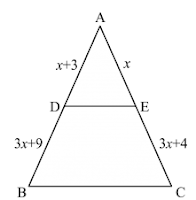Solution

Given : In △ABC , DE | | AB .
To find : the value x
According to basic proportionality theorem if a line is drawn parallel to one side of a triangle intersecting the other two sides, then it divides the two sides in the same ratio.
In △ABC , DE | | AB .
x+3/3x +19 = x/3x+4
(x+3)(3x+4) = (x)(3x+19)
3x2 + 4x + 9x + 12 = 3x2 + 19x
19x – 13x = 12
6x = 12
x = 2
Hence we got the result d .

33. In the given figure, if ∠ADE = ∠ABC, then CE =
(a) 2
(b) 5
(c) 9/2
(d) 3

Solution

To find: The value of CE
∴ DE | | BC (Two lines are parallel if the corresponding angles formed are equal)
According to basic proportionality theorem if a line is parallel to one side of a triangle intersecting the other two sides, then it divides the two sides in the same ratio.
2/3 = 3/EC
EC = 3×3/2
EC = 9/2
Hence we got the result c .

34. In the given figure, RS | | DB | | PQ. If CP = PD = 11 cm and DR = RA = 3 cm . Then the values of x and y are respectively .
(a) 12,10
(b) 14,6
(c) 10,7
(d) 16,8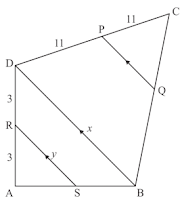Solution

Given : RS | | DB | | PQ . CP = PD = 11 cm and DR = RA = 3 cm
To find : the value of x and y respectively .
In △ASR and △ABD ,
∠ASR = ∠ABQ (Corresponding angles)
∠A = ∠A (Common)
∴ △ASR ~ △ABD (AA Similarity)
3/6 = RS/DB
1/2 = x/y
x = 2y
This relation is satisfied by option d.
Hence, x = 16 cm and y =8 cm
Hence the result is d .

35. In the given figure, if PB | | CF and DP | | EF, then AD/ DE =
(a) 3/4
(b) 1/3
(c) 1/4
(d) 2/3Solution

Given : PB | | CF and DP | | EF . AB = 2 cm and AC = 8 cm .
According to basic proportionality theorem, if a line is drawn parallel to one side of a triangle intersecting the other two sides . then it divides the two sides in the same ratio .
AB/BC = AP/PF
AP/PF = 2/8-2
AP/PF = 2/6
AP/PF = 1/3 …(1)
Again, DP | | EF .
Hence we got the result option b .

36. A chord of a circle of radius 10 cm subtends a right at the centre. The length of the chord (in cm) is
(a) 5 √2
(b) 10√2
(c) 5 /√2
(d) 105√3

SolutionIn right ΔOAB,
AB2 = OA2 + OB2 (Pythagoras theorem)
⇒ AB2 = (10)2 + (10)2 (OA = OB = 10 cm)
⇒ AB2 = 100 + 100 = 200
⇒ AB = √200 = 10√2 cm
Thus, the length of the chord is 10√2 cm
Hence the correct answer is option B.

37. A vertical stick 20 m long casts a shadow 10 m long on the ground . At the same time , a tower casts a shadow 50 m long on the ground . The height of the tower is
(a) 100 m
(b) 120 m
(c) 25 m
(d) 200 m

Solution

Given : Vertical stick 20 m long casts a shadow 10 m long on the ground . At the same time a tower casts the shadow 50 m long on the ground.
To determine : Height of the tower
Let AB be the vertical stick and AC be its shadow . Also , let DE be the vertical tower and DF be its shadow.
Join BC and EF.In ΔABC and ΔDEF , we have
∠A = ∠D = 90°
∠C = ∠F
ΔABC ~ ΔDEF
We know that in any two similar triangles , the corresponding sides are proportional . Hence ,
AB/DE = AC/DF
20/DE = 10/50
DE = 100 m
Hence the correct answer is option a .

38. Two isosceles triangles have equal angles and their areas are in the ratio 16:25. The ratio of their corresponding heights is
(a) 4:5
(b) 5:4
(c) 3:2
(d) 5:7

Solution

Given two isosceles triangle have equal vertical angles and their areas are in the ratio of 16:25 .
To find : ratio of their corresponding heights .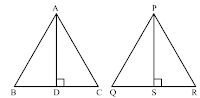Let ΔABC and ΔPQR be two isosceles triangles such that ∠A = ∠P . Suppose AD ⊥ BC and PS ⊥ QR .
In ΔABC and ΔPQR ,
AB/PQ = AC/PR
∠A = ∠P
∴ ΔABC ~ ΔPQR (SAS similarity)
We know that the ratio of areas of two similar triangles is equal to the ratio of squares of their corresponding altitudes .
Hence,
Hence we got the result as a .

39. ΔABC is such that AB = 3 cm , BC = 2 cm and CA = 2.5 cm . If ΔDEF ~ ΔABC and EF = 4 cm , then perimeter of ΔDEF is
(a) 7.5 cm
(b) 15 cm
(c) 22.5 cm
(d) 30 cm

Solution

Given : In ΔABC , AB = 3 cm, BC = 2 cm , CA = 2.5 cm . ΔDEF ~ ΔABC and EF = 4 cm
To find : Perimeter of ΔDEF .
We know that if two triangles are similar , then their sides are proportional
Since ΔABC and ΔDEF are similar ,
AB/DE = BC/EF = CA/FD
3/DE = 2/4 = 2.5/FD
3/DE = 2/4
DE = 6 cm …(1)
2/4 = 2.5/FD
FD = 5 cm …(2)
From (1) and (2) , we get
Perimeter of ΔDEF = DE + EF + FD = 6 + 4 + 5 = 15 cm
Hence the correct answer is b .

40. In ΔABC , a line XY parallel to BC cuts AB at X AC at Y . If BY bisects ∠XYC , then
(a) BC = CY
(b) BC = BY
(c) BC ≠ CY
(d) BC ≠ BY

Solution

Given XY | | BC and BY is bisector of ∠XYC .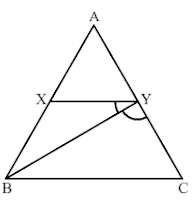Since XY | | BC
So ∠YBC = ∠BYC (Alternate angles)
Now, in triangles BYC two angles are equal . Therefore , the two corresponding sides will be equal
Hence, BC = CY
Hence option a is correct.

41. In a ΔABC , ∠A = 90°, AB = 5 cm and AC = 12 cm . If AD ⊥ BC , then AD =
(a) 13/2 cm
(b) 60/13 cm
(c) 13/60 cm
(d) 2√15/13 cm

Solution

Given : In ΔABC ∠A = 90°, AD ⊥ BC , AC = 12 cm, and AB = 5 cm .
We know that the ratio of areas of two similar triangles is equal to the ratio of squares of their corresponding sides .∠C = ∠C (Common)
∴ ΔACB ~ ΔADC (AA Similarity)
We got the result as b .

42. In a ΔABC, perpendicular AD from A and BC meets BC at D . If BD = 8 cm, DC = 2 cm and AD = 4 cm, then
(a) ΔABC is isosceles
(b) ΔABC is equilateral
(c) AC = 2AB
(d) ΔABC is right – angled at A

Solution

Given : In ΔABC, AD ⊥ BC , BD = 8 cm, DC = 2 cm and AD = 4 cm .

AC2 = 42 + 22
AC2 = 20 …..(1)
AC2 = 42 + 82
AB2 = 80 …..(2)
Now, in ΔABC
BC2 = (CD + DB)2 = (2+8)2 = (10)2 = 100
and
AB2 + CA2 = 80 + 20 = 100
∴ AB2 + CA2 = BC2
Hence, triangle ABC is right angled at A .
We got the result as d .

43 . In a ΔABC, point D is on side AB and point E is on side AC , such that BCED is a trapezium . If DE : BC = 3:5, then Area (ΔADE) : Area(ΔBCED) =
(a) 3:4
(b) 9:16
(c) 3:5
(d) 9:25

Solution

Given : In ΔABC,D is on side AB and point E is on side AC, such that BCED is a trapezium . DE : BC = 3:5.
To find : Calculate the ratio of the areas of ΔADE and the trapezium BCED .
∠A = ∠A (Common)
∴ ΔADE ~ ΔABC (AA Similarity) .We know that
Let Area of ΔADE = 9x sq. units and Area of ΔABC = 25x sq. units
Ar[trap BCED] = Ar(ΔABC) – Ar(ΔADE)
= 25x – 9x
= 16x sq units
Now,
Hence the correct answer is b .

44. If ABC is an isosceles triangle and D is a point of BC such that AD ⊥ BC , then
(a) AB2 - AD2 = BD.DC
(b) AB2 – AD2 = BD2 – DC2
(c) AB2 + AD2 = BD.DC
(d) AB2 + AD2 = BD2 – DC2

Solution

Given : ΔABC is an isosceles triangle , D is a point on BC such that AD ⊥ BC
We know that in an isosceles triangle the perpendicular from the vertex bisects the Base .
∴ BD = DCApplying Pythagoras theorem in ΔABD
⇒ AB2 – AD2 = BD × BD
Since BD = DC
⇒ AB2 – AD2 = BD × BD
Since BD = DC
⇒ AB2 – AD2 = BD × BD
Hence correct answer is a .

45 . ΔABC is a right triangle right – angled at A and AD ⊥ BC . Then , BD/DC =
(a) (AB/AC)2
(b) AB/AC

Solution

Given : In ΔABC, ∠A = 90° and AD ⊥ BC .
To find : BD : DCFrom (1) and (2),
Disclaimer : The question is not correct . The given ratio cannot be evaluated using he given conditions in the questions .

46. If E is a point on side CA of an equilateral triangle ABC such that BE ⊥ CA, then AB2 + BC2 + CA2 =
(a)2 BE2
(b) 3 BE2
(c) 4 BE2
(d) 6 BE2

Solution

In triangle ABC , E is a point on AC such that BE ⊥ AC .
We need to find AB2 + BC2 + AC2.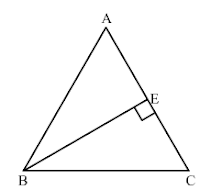Since BE ⊥ AC , CE = AE = AC/2 (In a equilateral triangle, the perpendicular from the vertex bisects the base.
In triangle ABE, we haveAB2 = BE2 + AE2
Since AB = BC = AC
Therefore , AB2 = BC2 = AC2 = BE2 + AE2
⇒ AB2 + BC2 + AC2 = 3BE2 + 3AE2
Since in triangle BE is an altitude, so BE = √3/2 AB
BE = √3/2 AB
= √3/2 × AC
= √3/2 × 2AE = √3AE
⇒ AB2 + BC2 + AC2 = 3BE2 + 3(BE/√3)2
= 3BE2 + BE2 = 4BE2
Hence option c is correct .

47. In a right triangle ABC right – angled at B, if P and Q are points on the sides AB and AC respectively , then

(a) AQ2 + CP2 = 2(AC2 + PQ2)
(b) 2(AQ2 + CP2) = AC2 + PQ2
(c) AQ2 + CP2 = AC2 + PQ2
(d) AQ + CP = 1/2 (AC + PQ)

Solution

Disclaimer : There is mistake in the problem . The question should be ‘’ In a right triangle ABC right – angled at B, if P and Q are points on the sides AB and BC respectively , then ‘’
Given : In the right △ABC , right angled at B. P and Q are points on the sides AB and BC respectively .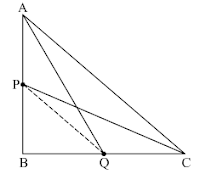Applying Pythagoras theorem,
In △AQB,
AQ2 = AB2 + BQ2 …(1)
In △PBC
CP2 = PB2 + BC2 …(2)
Adding (1) and (2), we get
AQ2 + CP2 = AB2 + BQ2 + PB2 + BC2
…(3)
In △ABC ,
AC2 = AB2 + BC2 …(4)
In △PBQ ,
PQ2 = PB2 + BQ2 …(5)
From (3), (4) and (5) , we get
AQ2 + CP2 = AC2 + PQ2
We got the result as c .

48. If △ABC ~ △DEF such that DE = 3 cm, EF = 2 cm, DF = 2.5 cm, BC = 4 cm, then perimeter of △ABC is
(a) 18 cm
(b) 20 cm
(c) 12 cm
(d) 15 cm

Solution

Given : △ABC and △DEF are similar triangles such that DE = 3 cm, EF = 2 cm, DF = 2.5 cm and BC = 4 cm .
To find : Perimeter of △ABC .
We know that if two triangles are similar then their corresponding sides are proportional .
Hence , AB/DE = BC/EF = CA/FD
Substituting the values we get
AB/BC = DE/EF
AB/4 = 3/2
AB = 6 cm …(1)
Similarly,
CA/BC = DF/EF
CA/4 = 2.5/2
CA = 5 cm …(2)
Perimeter of △ABC = AB + BC + CA
= 6+4+5
= 15 cm
Hence the correct option is d .

49. In △ABC ~ △DEF such that AB = 9.1 cm and DE = 6.5 cm . If the perimeter of △DEF is 25 cm , then the perimeter of △ABC is
(a) 36 cm
(b) 30 cm
(c) 34 cm
(d) 35 cm

Solution

Given △ABC is similar to △DEF such that AB = 9.1 cm , DE = 6.5 cm . Perimeter of △DEF is 25 cm .To find : Perimeter of △ABC .
We know that the ratio of corresponding sides of similar triangles is equal to the ratio of their perimeters .
AB/DE = BC/EF = AC/DE = P1/P2
AB/DE = P(△ABC )/P(△DEF)
9.1/6.5 = P(△ABC )/25
P(△ABC ) = 9.1×25/6.5
P(△ABC ) = 35 cm
Hence the correct answer is d .

50. In an isosceles triangle ABC if AC = BC and AB2 = 2AC2, then ∠C =
(a) 30°
(b) 45°
(c) 90°
(d) 60°

Solution

Given : In Isosceles △ABC , AC = BC and AB2 = 2AC2 .
To find : Measure of angle C
In isosceles △ABC ,
AC = BC
∠B = ∠A (Equal sides have equal angles opposite to them )AB2 = 2AC2
AB2 = AC2 + AC2
AB2 = AC2 + BC2 (AC = BC)
⇒ △ABC is right angle triangle , with ∠C = 90°
Hence the correct answer is c.